# SAT II Math I : Analyzing Figures

## Example Questions

### Example Question #1 : Analyzing Figures

The sides of a triangle have lengths 6 yards, 18 feet, and 216 inches. Which of the following is true about this triangle?

The triangle is acute and equilateral.

This triangle is acute and isosceles, but not equilateral.

This triangle is right and scalene.

This triangle is acute and scalene.

This triangle is right and isosceles, but not equilateral.

The triangle is acute and equilateral.

Explanation:

One yard is equal to 3 feet; it is also equal to 36 inches. Therefore:

18 feet is equal to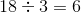yards,

and

216 feet is equal to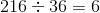yards.

The three sides are congruent, making the triangle equilateral - and all equilateral triangles are acute.

### Example Question #2 : Analyzing Figures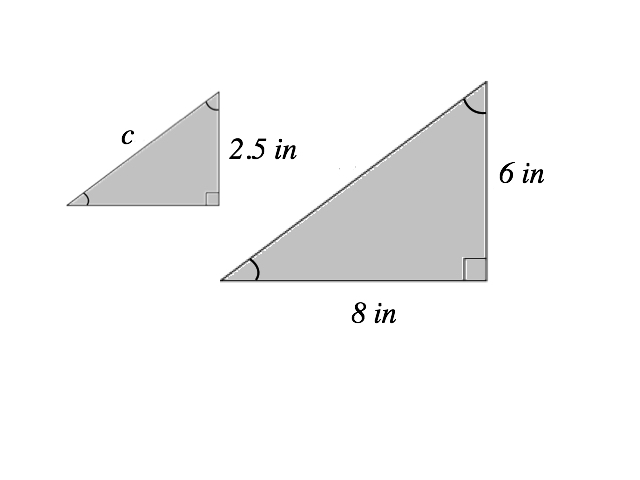Figures not drawn to scale

# The triangles above are similar. Given the measurements above, what is the length of side c?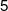inches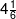inches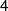inches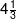inches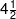inchesinches

Explanation:

You can find the length of c by first finding the length of the hypotenuse of the larger similar triangle and then setting up a ratio to find the hypotenuse of the smaller similar triangle.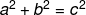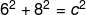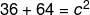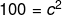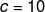You also could have found 10 by recognizing this triangle is a form of a 3-4-5 triangle.

### The hypotenuse of the bigger triangle is 10 inches.

Now that we know the length of the hypotenuse for the larger triangle, we can set up a ratio equation to find the hypotenuse of the smaller triangle.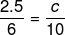cross multiply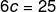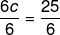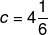### Example Question #2 : Analyzing Figures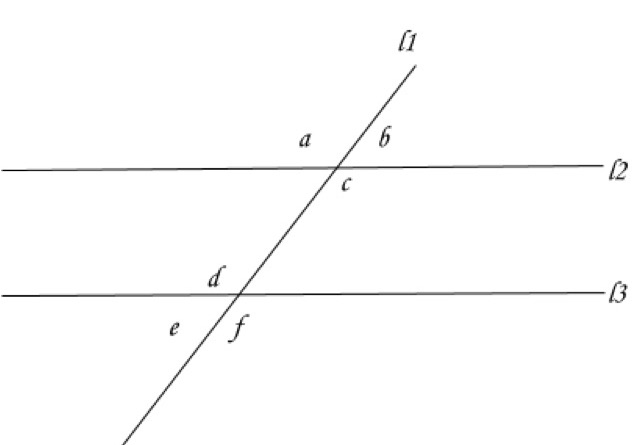If line 2 and line 3 eventually intersect when extended to the left which of the following could be true?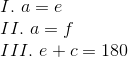I and III

Cannot be determined

I, II, and III

I only

I and II

I only

Explanation:

Read the question carefully and notice that the image is deceptive: these lines are not parallel. So we cannot apply any of our rules about parallel lines. So we cannot infer II or III, those are only true if the lines are parallel. If we sketch line 2 and line 3 meeting we will form a triangle and it is possible to make a = e. One such solution is to make a and e 60 degrees.

### Example Question #3 : Analyzing Figures

What is the maximum number of distinct regions that can be created with 4 intersecting circles on a plane?Explanation:

Try sketching it out.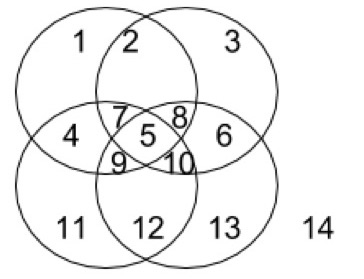Start with one circle and then keep adding circles like a venn diagram and start counting. A region is any portion of the figure that can be defined and has a boundary with another portion. Don't forget that the exterior (labeled 14) is a region that does not have exterior boundaries.

### Example Question #72 : 2 Dimensional Geometry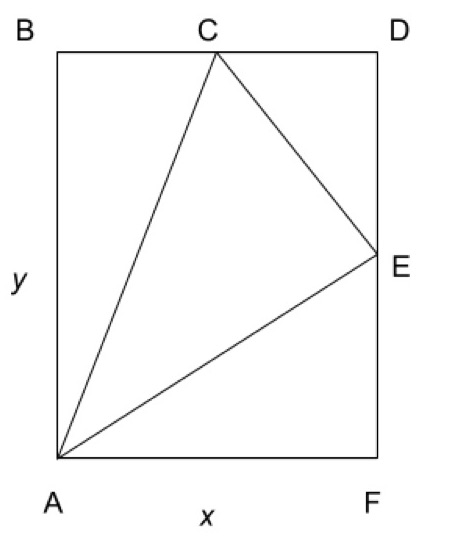Note: Figure may not be drawn to scale

In rectangle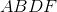has length and widthandrespectively. Pointlies on line segment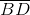and point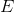lies on line segment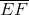.  Triangle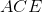has area, in terms ofandwhat is the possible range of values for?

cannot be determined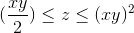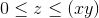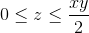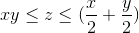Explanation:

Notice that the figure may not be to scale, and pointsandcould lie anywhere on line segmentsandrespectively.

Next, recall the formula for the area of a triangle: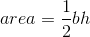To find the minimum area we need the smallest possible values forand.

To makesmaller we can shift pointsandall the way to point. This will make trianglehave a height of: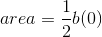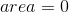is the minimum possible value for the area.

To find the maximum value we need the largest possible values forand. If we shift pointall the way to pointthen the base of the triangle isand the height is, which we can plug into the formula for the area of a triangle: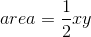which is the maximum possible area of triangle